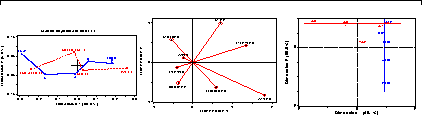# Chapter 5. Correspondence analysisCorrespondence analysis provides visualizations of associations in a two-way contingency table in a small number of dimensions. Multiple correspondence analysis extends this technique to n-way tables. Other grahical methods, including mosaic matrices and biplots provide complementary views of loglinear models for two-way and n-way contingency tables. 5.1. Simple correspondence analysis 5.1.1. Notation and terminology 5.1.2. Geometric and statistical properties 5.1.3. The CORRESP Procedure 5.1.4. The CORRESP macro 5.1.5. Quasi-independence and structural zeros 5.2. Properties of category scores 5.2.1. Optimal category scores 5.2.2. Simultaneous linear regressions 5.3. Multi-way tables 5.3.1. Marginal tables and supplementary variables 5.4. Multiple correspondence analysis 5.4.1. Bivariate MCA 5.4.2. The Burt matrix 5.4.3. Multivariate MCA 5.5. Extended MCA: Showing interactions in 2Q tables 5.6. Biplots for contingency tables 5.6.1. Biplots for two-way tables 5.6.2. Biplots for three-way tables 5.7. Chapter summary